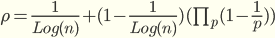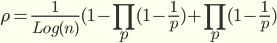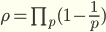# Calculating the coprime probability of two integers in a different way

The probability that two randomly chosen integers to be coprimes is known to be equal to ## \prod_{2}^{\infty}(1-\frac{1}{p^2})=6/\pi^2##​
I tried to conceptualize the problem in the following way but got different results.
Suppose that we pick up an integer at random which could be either prime or composite. Let's pick up another integer smaller than the first one and wonder about the probability of both integers being coprimes.
##\rho=(P_p)(cop|P_p)+(not P_p)(cop|not P_p)##

Where ##\rho## is the probability that the two integers are coprimes which can be expressed as the sum of the probability that the first integer is a prime multiplied by the conditional probability of the second integer to be coprime given that the first one is prime plus the probability that the first integer being a non-prime multiplied by the conditional probability of the second number to be coprime given the first one is non-prime.
Since we know the frequency of the prime from the PNT (prime number theorem) we can substitute that for the probability of the prime.
Also, from the Euler Phi function, we can substitute it for the last term. ##\varphi=\prod_{p}^{} n(1-\frac{1}{p})##

we get:
##\rho=\frac{1}{Log(n)}+(1-\frac{1}{Log(n)})(\frac{\prod_{p}^{} n(1-\frac{1}{p})}{n})##
n cancel and we left up withAfter factoring out we getFor a large n ##\frac{1}{Log(n)}## goes to zero

and the final result becomeswhich differs from the correct one by a power of 2 for P.

Last edited:

Gold Member
The probability that two randomly chosen integers to be coprimes is known to be equal to
Thanks for interest information. Now I know how to get to the formula as follows.

Probability of both the numbers are even is ##1/2^2##
So the probability of its complement is ##1-1/2^2##

Probability of both the numbers are multiple of 3 is ##1/3^2##
So the probability of its complement is ##1-1/3^2##

Probability of both the numbers are multiple of 5 is ##1/5^2##
So the probability of its complement is ##1-1/5^2##

So and so. The product of all the complements are the formula whose value is approx. and less than 0.61. Product to infinity suggests that the numbers should have upper limit. If the numbers have upper limit we would have higher probability than ##6/\pi^2## because p for product must be less than the numbers.

Last edited:
Mentor
##\rho=\prod_{p}^{} (1-\frac{1}{p})## is the result for a specific large number where you only take the product over the prime factors of that large number. You didn't consider the probability that a given prime number is included in the product, which is another factor 1/p.

Note that there is no uniform distribution over the integers, so you need to define some limit process. Choosing a specific n will never give the right answer anyway.

•pbuk
You didn't consider the probability that a given prime number is included in the product, which is another factor 1/p.

Mentor
Your product doesn't go over all primes. It will include the factor 1-1/2 only for half of the numbers (the even ones). It will include the factor 1-1/3 only for 1/3 of the numbers (numbers divisible by 3). And so on.

Instead of subtracting 1/2 you should subtract 1/2 only in 1/2 of the cases.
Instead of subtracting 1/3 you should subtract 1/3 only in 1/3 of the cases.
All these factors become 1-1/p2 after taking this into account.

•pbuk
Gold Member
I delete this comment.

Last edited:
Gold Member
and the final result becomeswhich differs from the correct one by a power of 2 for P.
I observe ρ is probability that a random chosen number with no upper limit is a prime number other than prime numbers accounted from 2 to infinity. So it is zero. Actually

$$\log \ \rho = \sum \log(1-1/p)=-\sum 1/p + ... =-\infty$$

Last edited:
Homework Helper
Gold Member
I observe ρ is probability that a random chosen number with no upper limit is a prime number other than prime numbers accounted from 2 to infinity.
I find it impossible to understand what you are trying to say. What are 'prime numbers accounted from 2 to infinity'? Which parts of that sentence are supposed to go with which other parts? It is meaningless to talk about 'a random chosen number with no upper limit': as @mfb said:
there is no uniform distribution over the integers, so you need to define some limit process

Gold Member
@pbuk
So may I understand that the statement at the beginning of OP
The probability that two randomly chosen integers to be coprimes is known to be equal to ∏2∞(1−1p2)=6/π2I
is meaningless because it is talking about random chosen numbers with no upper limit ?
Do you have an estimation of value of ##\rho## other than zero ?

I think that for random chosen N >>P where P is a prime number,
$$\sum_{p=2}^P \log(1-1/p)$$
shows an approximate probability that N is coprime with prime numbers from 2 to P.
Taking limit of both N and P keeping N >> P , I hope it becomes as I said in #7.

Last edited:
Mentor
The statement is good but it needs to be understood as a limit. The probability that two uniformly random chosen numbers from 1 to N is coprime goes to that expression in the limit of N->infinity.

As neither number is fixed we can't have an expression that takes a sum or product based on the divisibility of a specific number.
shows an approximate probability that N is coprime with prime numbers from 2 to P.
Yes, and it shows the fraction of primes up to N goes to zero for N->infinity, but that's not what OP asked about.

Gold Member
So we together would regret to say to OP that his formula of ##\rho## has nothing to do with the problem he raised and it is zero.

Last edited:
Homework Helper
Gold Member
So may I understand that the statement at the beginning of OP is meaningless because it is talking about random chosen numbers with no upper limit ?
No it is not meaningless, but to understand it you need to insert some words:

The [limit of the] probability that two randomly chosen integers [in a domain tending to infinity] to be coprimes is known to be equal to ## \prod_{2}^{\infty}(1-\frac{1}{p^2})=6/\pi^2 ##.

Do you have an estimation of value of ##\rho## other than zero ?
No I don't need an estimation: there is a concise proof of that statement repeated all over the internet: WikiPedia, Math StackExchange etc...

When an OP starts a topic by saying that something is 'known' that is not known to you then it is a good idea to go and find out about the topic rather than derail the thread by trying to disprove it.

•mfb and anuttarasammyak
Homework Helper
Gold Member
So we together would regret to say to OP that his formula of ##\rho## has nothing to do with the problem he raised and it is zero.
You should not decide what anyone else would do together with you.

Read the OP again. It can be summarised like this:
• I know that ## y = f(x) ## can be proved.
• Using the reasoning below I get the result ## y = g(x), g \ne f##.
• Where did I go wrong?
It does not help anyone to ignore this completely and come up with some poorly researched workings that attempt to show that ## y = h(x) ##.

•anuttarasammyak
Gold Member
Thanks.

I try an inverse way of OP, starting from element in ##\rho## which says about one number and a prime number are coprime, to ##6/\pi^2## which says about two numbers are coprime.

For two numbers A and B
Probabilities for the cases A and B are coprime:
A and p are coprime, B and p are coprime : ## (1-1/p)(1-1/p) ##
A and p are coprime, B is multiple of p : ## (1-1/p) 1/p ##
B and p are coprime, A is multiple of p : ## (1-1/p) 1/p ##
----------------------------
Sum ## (1-1/p^2)##

Making the product for all prime numbers p we get ##6/\pi^2##.
Here factor (1-1/p) which consists of ##\rho## tells that we are always thinking that at least one number and a prime number are coprime.

Last edited:
•